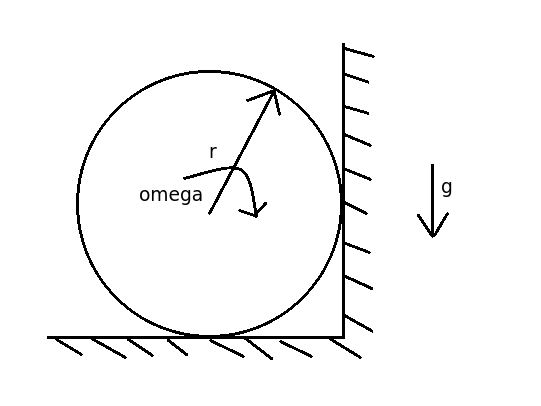# Cylinder's back against the wall

Rotating cylinders are not just physical abstractions: for example, they can turn a flow of the air, thus creating lift as the airplane wings. Historically people have even used rotating cylinders to power ships (although with little efficiency). NASA wrote more about this here.

We will now examine how much time we need to stop a ship-engine-like cylinder. Suppose you take a rotating cylinder and let it roll to the right until it reaches a vertical wall. It then spins against the two walls as shown in the picture, slowly slowing down. How much time will it take in s for the cylinder to stop?Details and assumptions

• The initial speed of the cylinder's surface when it touches the vertical wall is $30~\mbox{m/s}$.
• Its length is $l = 10~\mbox{m}$, its radius is $r = 0.5~\mbox{m}$ and it's made out of wood of density $\rho = 800~\mbox{kg/m}^3$.
• The coefficient of friction between the cylinder and the walls is $\mu = 0.3$.
• The acceleration of gravity is $-9.8~\mbox{m/s}^2$.
• There are at least two ways to approach this problem.
×

Problem Loading...

Note Loading...

Set Loading...